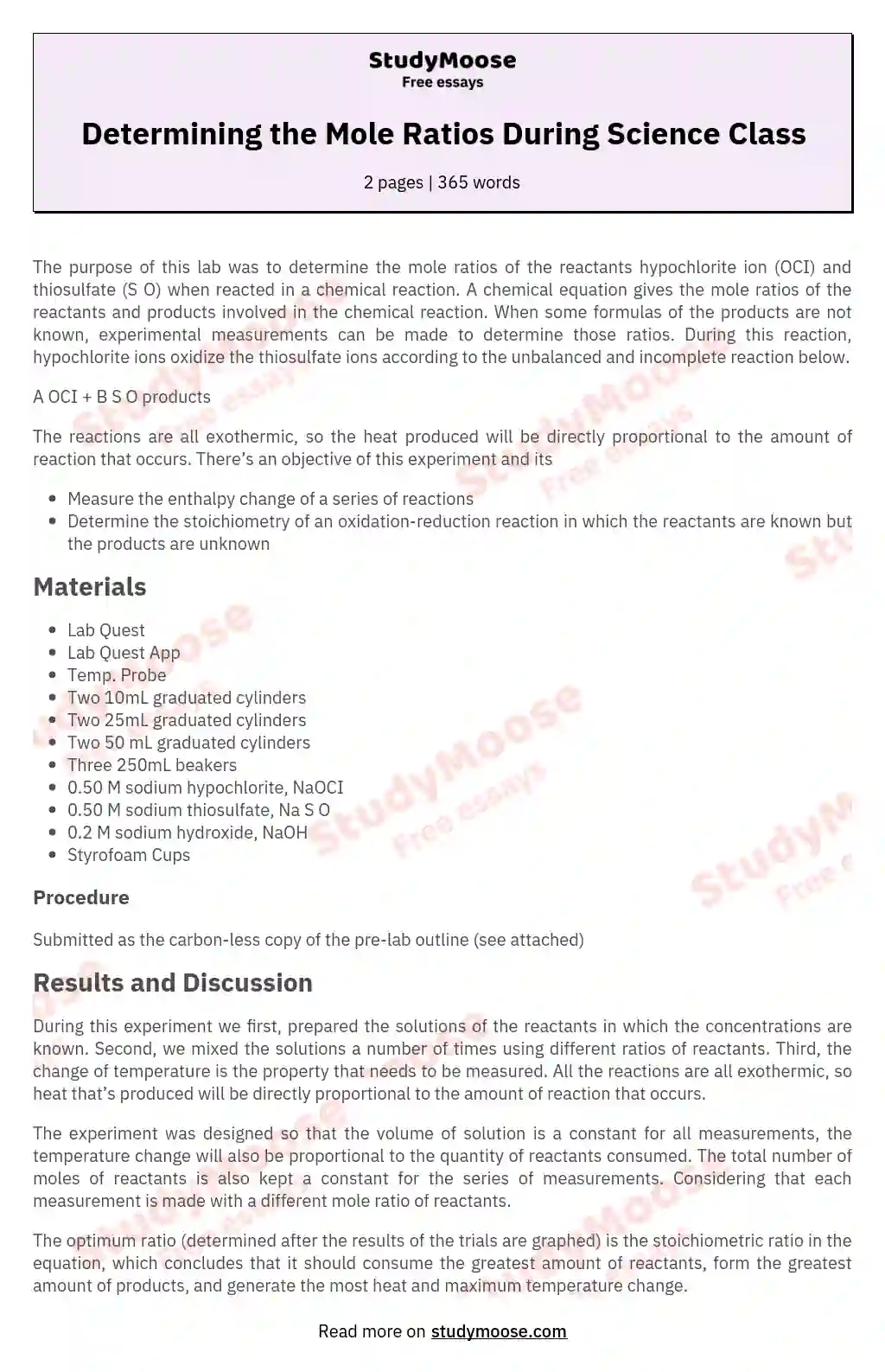# Determining the Mole Ratios During Science Class

Categories: Science

The purpose of this lab was to determine the mole ratios of the reactants hypochlorite ion (OCI) and thiosulfate (S O) when reacted in a chemical reaction. A chemical equation gives the mole ratios of the reactants and products involved in the chemical reaction. When some formulas of the products are not known, experimental measurements can be made to determine those ratios. During this reaction, hypochlorite ions oxidize the thiosulfate ions according to the unbalanced and incomplete reaction below.

A OCI + B S O products

The reactions are all exothermic, so the heat produced will be directly proportional to the amount of reaction that occurs.

Get to Know The Price Estimate For Your Paper
Topic
Number of pages
Email Invalid email

You won’t be charged yet!

There’s an objective of this experiment and its

• Measure the enthalpy change of a series of reactions
• Determine the stoichiometry of an oxidation-reduction reaction in which the reactants are known but the products are unknown

## Materials

• Lab Quest
• Lab Quest App
• Temp. Probe
• Two 50 mL graduated cylinders
• Three 250mL beakers
• 0.50 M sodium hypochlorite, NaOCI
• 0.50 M sodium thiosulfate, Na S O
• 0.2 M sodium hydroxide, NaOH
• Styrofoam Cups

### Procedure

Submitted as the carbon-less copy of the pre-lab outline (see attached)

## Results and Discussion

During this experiment we first, prepared the solutions of the reactants in which the concentrations are known. Second, we mixed the solutions a number of times using different ratios of reactants. Third, the change of temperature is the property that needs to be measured. All the reactions are all exothermic, so heat that’s produced will be directly proportional to the amount of reaction that occurs.

The experiment was designed so that the volume of solution is a constant for all measurements, the temperature change will also be proportional to the quantity of reactants consumed. The total number of moles of reactants is also kept a constant for the series of measurements. Considering that each measurement is made with a different mole ratio of reactants.

The optimum ratio (determined after the results of the trials are graphed) is the stoichiometric ratio in the equation, which concludes that it should consume the greatest amount of reactants, form the greatest amount of products, and generate the most heat and maximum temperature change.